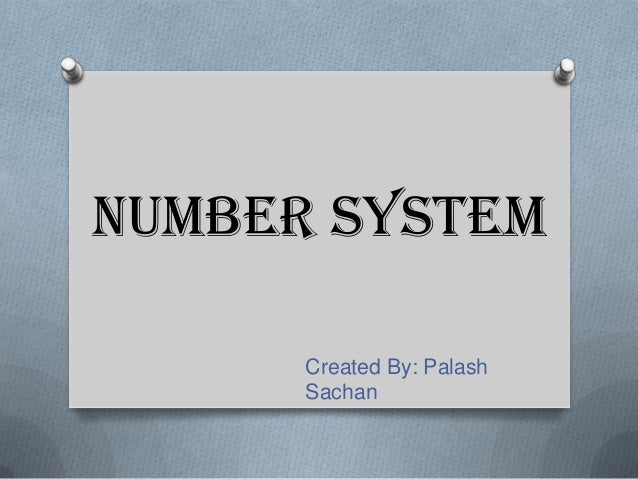Successfully reported this slideshow.Upcoming SlideShare
×

# Number system

32,792 views

Published on

http://www29.zippyshare.com/v/42478054/file.html

Number System
Decimal Number System
Binary Number System
Why Binary?
Octal Number System
Relationship between Hexadecimal, Octal, Decimal, and Binary
Number Conversions

Published in: Education, Technology
• Full Name
Comment goes here.

Are you sure you want to Yes No### Number system

1. 1. Number SystemCreated By: PalashSachan
2. 2. CONTENTS Introduction Decimal Number System Binary Number System Why Binary? Octal Number System Hexadecimal Number System Relationship between Hexadecimal, Octal,Decimal, and Binary Number Conversions
3. 3. INTRODUCTIONIn early days when there were no means ofcounting, people use to count with the help offingers, stones, sticks, etc.These methods were not adequate and had manylimitations.Many number system were introduced with thepassage of time like: Decimal Number System Binary Number System Octal Number System Hexadecimal Number System
4. 4. Decimal Number System• It consist of ten digit i.e. 0, 1, 2, 3, 4, 5, 6, 7, 8,9 with the base 10.• Each number can be used individually or theycan be grouped to form a numeric value as85,48,35,456 etc.
5. 5. BINARY NUMBER SYSTEM• The Binary Number System consist of only twodigits– 0 and 1.• Since this system use two digits, it has thebase 2.• All digital computer use this number systemand convert the data input from the decimalformat into its binary equivalent.
6. 6. Why Binary?Since the computer is made up of electroniccomponents; it can have only two states, either• On(1)• Off(0)The data which is given to the computer isconverted into binary form because a computerunderstand only binary language.It further converts the binary results into theirdecimal equivalents for output.
7. 7. Octal Number System In the Octal Number System it consist of 8digits i.e. 0, 1, 2, 3, 4, 5, 6, 7 with a base 8. The sequence of octal number goes as 0, 1, 2,3, 4, 5, 6, 7, 10, 11, 12, 13, 14, 15, 16, 17, 20,21, 22, …..as go on. See each successive number after 7 is acombination of two or more unique symbols ofoctal system.
8. 8. Hexadecimal Number System The Hexadecimal system use base 16. It has 16 possible digit symbol. It use the digit 0 through 9 plus the letters A, B,C, D, E, and F as the 16 digit symbols.
9. 9. Relationship between Hexadecimal, Octal,Decimal, and Binary Notice that eachhexadecimal digitrepresent agroup of fourbinary digit. It Isimportant toremember thatHex(Abbreviationfor Hexadecimal)digit A through Fare equivalent tothe decimal value10 through 15.HexadecimalOctal Decimal Binary0 0 0 00001 1 1 00012 2 2 00103 3 3 00114 4 4 01005 5 5 01016 6 6 01107 7 7 01118 10 8 10009 11 9 1001A 12 10 1010B 13 11 1011C 14 12 1100D 15 13 1101E 16 14 1110F 17 15 1111
10. 10. NUMBER CONVERSIONS
11. 11. Decimal-to-Binary ConversionThe method of converting Decimal to binary isrepeated-division method. For conversion followthe rules:1. Divide the given decimal number with thebase 2.2. Write down the remainder and divide thequotient by 2.3. Repeat step 2 till the quotient is zero.
12. 12. 2 200 Remainders2 100 0 LSB2 50 02 25 0Write2 12 1 in2 6 0 this2 3 0order2 1 10 1 MSB
13. 13. Binary-to-Decimal ConversionTo convert a binary number follow the steps:1. Multiply each binary number with 2 having thepower 0 for last position, starting from the rightdigit.2. Increase the power one by one, with base as2.3. Sum up all the products to get decimalnumber.
14. 14. Decimal-to-OctalThe method of converting Decimal to Octal isrepeated-division method. For conversion followthe rules:1. Divide the given decimal number with thebase 8,2. Write down the remainder and divide thequotient by 8,3. Repeat step 2 till the quotient is zero.
15. 15. 8 266 Remainders8 33 2 LSB8 4 10 4MSB
16. 16. Octal-to-Decimal ConversionTo convert a octal number follow the steps:1. Multiply each binary number with 8 having thepower 0 for last position, starting from the rightdigit.2. Increase the power one by one, with base as8.3. Sum up all the products to get decimalnumber.
17. 17. Octal-to-Binary ConversionThe conversion from octal to binary is performedby converting each octal digit to its 3-bit binaryequivalent.The eight possible digits are converted asindicated below:Using these conversions, any octal number isconverted to binary by individually convertingeach digit.Octal Digit 0 1 2 3 4 5 6 7BinaryEquivalent000 001 010 011 100 101 110 111
18. 18. Binary-to-Octal Conversion
19. 19. Decimal-to-Hexadecimal ConversionThe method of converting Decimal to binary isrepeated-division method. For conversion followthe rules:1. Divide the given decimal number with thebase 16.2. Write down the remainder and divide thequotient by 16.3. Repeat step 2 till the quotient is zero.
20. 20. 16 423 Remainders16 26 7 LSB16 1 A0 1 MSB
21. 21. Hexadecimal-to-Decimal ConversionTo convert a binary number follow the steps:1. Multiply each binary number with 16 havingthe power 0 for last position, starting from theright digit.2. Increase the power one by one, with base as16.3. Sum up all the products to get decimalnumber.